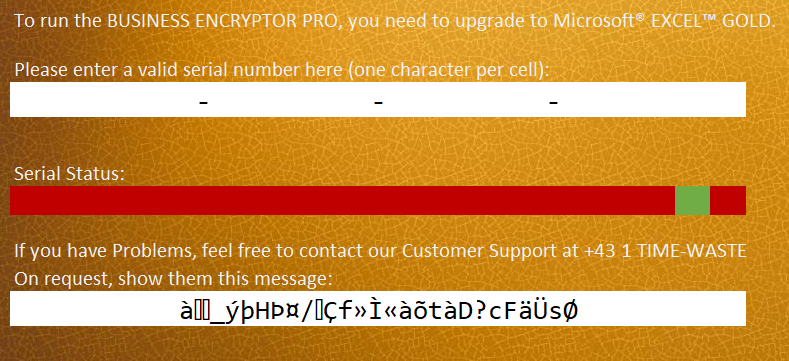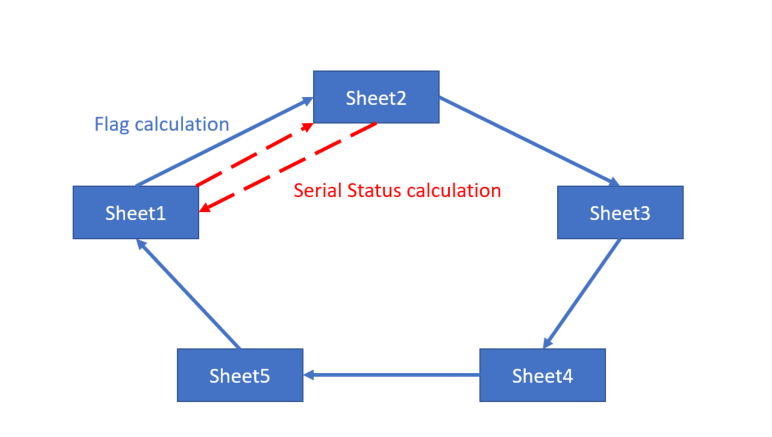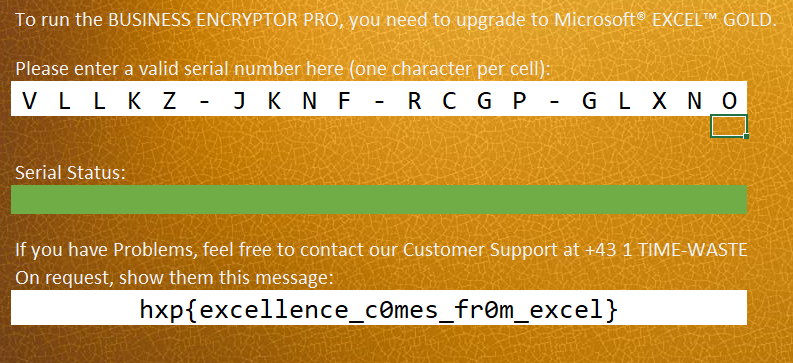# 0xd13a

A rookie in a world of pwns

# HXP CTF 2020 Writeup: EXCELlent

EXCELlent

Excellent CTFs need excellent business strategies, and what would be more appropriate than Microsoft ® Excel ™?

Of course, you need the excellent Excel Gold subscription to be as excellent as hxp. But of course, that won’t be a problem for all the excellent hackers like you.

This was an interesting old-school challenge. When I opened the Excel file I expected tricky obfuscated scripting, VBA stomping, or the like, but it was just a simple spreadsheet with a bunch of formulas. Actually a lot of formulas.

The front sheet asks for a serial number, and as you change it, portions of the “Serial Status” bar change color. Presumably when the whole bar is green the message in the bottom will display the flag:Let’s open the file in something like 7Zip. There are 5 sheets defined as XML files under `xl/worksheets`. The formulas inside connect different sheets together. Some formulas are involved in calculating Serial Status and others are used to calculate the flag. Let’s plot the relationship:Note that Serial Status calculation is pretty localized, we could just analyze that and once we figure out how to calculate the “green” status correctly we can find the serial number and then plug it in to get the flag.

Essentially all calculations we need are in Sheet2. Their number is small enough for us to analyze and iteratively fold and reduce them to the point that they only depend on the characters from the serial number. We can then use the z3 solver to find the serial.

Let’s put the reduced calculations in a script:

``````1
2
3
4
5
6
7
8
9
10
11
12
13
14
15
16
17
18
19
20
21
22
23
24
25
26
27
28
29
30
31
32
33
34
35
36
37
38
39
40
41
42
43
44
45
46
47
48
49
50
51
52
53
54
55
56
57
58
59
60
61
62
63
64
65
66
67
68
69
70
71
72
73
74
75
76
from z3 import *

size = 21

solver = Solver()

for i in range(size):
globals()['F%i' % i] = BitVec('F%i' % i, 32)
solver.add(And(globals()['F%i' % i] >= 0, globals()['F%i' % i] <= 255))

# Reduced expressions from Sheet2

solver.add(133 == ((255 & (globals()['F3'] >> 2)) | (255 & (globals()['F3'] << 6))) ^
((255 & (globals()['F20'] >> 1)) | (255 & (globals()['F20'] << 7))) ^
(((255 & (globals()['F11'] >> 1)) | (255 & (globals()['F11'] << 7))) ^
((255 & (globals()['F12'] >> 2)) | (255 & (globals()['F12'] << 6)))))
solver.add(225 == ((255 & (globals()['F4'] >> 3)) | (255 & (globals()['F4'] << 5))) ^
((255 & (globals()['F19'] >> 0)) | (255 & (globals()['F19'] << 8))) ^
(((255 & (globals()['F10'] >> 0)) | (255 & (globals()['F10'] << 8))) ^
((255 & (globals()['F13'] >> 3)) | (255 & (globals()['F13'] << 5)))))
solver.add(163 == ((255 & (globals()['F5'] >> 4)) | (255 & (globals()['F5'] << 4))) ^
((255 & (globals()['F18'] >> 8)) | (255 & (globals()['F18'] << 0))) ^
(((255 & (globals()['F9'] >> 8)) | (255 & (globals()['F9'] << 0))) ^
((255 & (globals()['F14'] >> 4)) | (255 & (globals()['F14'] << 4)))))
solver.add(212 == ((255 & (globals()['F6'] >> 5)) | (255 & (globals()['F6'] << 3))) ^
((255 & (globals()['F17'] >> 7)) | (255 & (globals()['F17'] << 1))) ^
(((255 & (globals()['F8'] >> 7)) | (255 & (globals()['F8'] << 1))) ^
((255 & (globals()['F15'] >> 5)) | (255 & (globals()['F15'] << 3)))))
solver.add(81 == (((((0+((globals()['F3'] ^ 17) >> 2)) % 256 + ((globals()['F4'] ^ 34) >> 2)) % 256 +
((globals()['F5'] ^ 51) >> 2)) % 256 + ((globals()['F6'] ^ 68) >> 2)) % 256 +
((globals()['F7'] ^ 85) >> 2)) % 256) ###
solver.add(248 == (((((0+((globals()['F3'] ^ 85) << 2)) % 256 + ((globals()['F4'] ^ 68) << 2)) % 256 +
((globals()['F5'] ^ 51) << 2)) % 256 + ((globals()['F6'] ^ 34) << 2)) % 256 +
((globals()['F7'] ^ 17) << 2)) % 256)
solver.add(116 == ((255 & (globals()['F7'] >> 6)) | (255 & (globals()['F7'] << 2))) ^
((255 & (globals()['F16'] >> 6)) | (255 & (globals()['F16'] << 2))))
solver.add(79 == ((((0+((globals()['F8'] ^ 17) >> 2)) % 256 + ((globals()['F9'] ^ 34) >> 2)) % 256 +
((globals()['F10'] ^ 51) >> 2)) % 256 + ((globals()['F11'] ^ 68) >> 2)) % 256)
solver.add(36 == ((((0+((globals()['F8'] ^ 68) << 2)) % 256 + ((globals()['F9'] ^ 51) << 2)) % 256 +
((globals()['F10'] ^ 34) << 2)) % 256 + ((globals()['F11'] ^ 17) << 2)) % 256)
solver.add(74  == ((((0+((globals()['F12'] ^ 17) >> 2)) % 256 + ((globals()['F13'] ^ 34) >> 2)) % 256 +
((globals()['F14'] ^ 51) >> 2)) % 256 + ((globals()['F15'] ^ 68) >> 2)) % 256)
solver.add(176 == ((((0+((globals()['F12'] ^ 68) << 2)) % 256 + ((globals()['F13'] ^ 51) << 2)) % 256 +
((globals()['F14'] ^ 34) << 2)) % 256 + ((globals()['F15'] ^ 17) << 2)) % 256)
solver.add(82 == (((((0+((globals()['F16'] ^ 17) >> 2)) % 256 + ((globals()['F17'] ^ 34) >> 2)) % 256 +
((globals()['F18'] ^ 51) >> 2)) % 256 + ((globals()['F19'] ^ 68) >> 2)) % 256 + ((globals()['F20'] ^ 85) >> 2)) % 256)
solver.add(60 == (((((0+((globals()['F16'] ^ 85) << 2)) % 256 + ((globals()['F17'] ^ 68) << 2)) % 256 +
((globals()['F18'] ^ 51) << 2)) % 256 + ((globals()['F19'] ^ 34) << 2)) % 256 + ((globals()['F20'] ^ 17) << 2)) % 256)
solver.add(0 == (globals()['F3'] & 32) + (globals()['F4'] & 32) + (globals()['F5'] & 32) +
(globals()['F6'] & 32) + (globals()['F7'] & 32) + (globals()['F8'] & 32) + (globals()['F9'] & 32) +
(globals()['F10'] & 32) + (globals()['F11'] & 32) + (globals()['F12'] & 32) + (globals()['F13'] & 32) +
(globals()['F14'] & 32) + (globals()['F15'] & 32) + (globals()['F16'] & 32) + (globals()['F17'] & 32) +
(globals()['F18'] & 32) + (globals()['F19'] & 32) + (globals()['F20'] & 32))
globals()['F8']-globals()['F9']-globals()['F10']-globals()['F11']+globals()['F12']+globals()['F13']+
globals()['F14']+globals()['F15']-globals()['F16']-globals()['F17']-globals()['F18']-globals()['F19']-
globals()['F20'])

# Find the solution

modl = solver.model()

# Collect the key characters from the solution

res = ""
for i in range(size):
obj = globals()['F%i' % i]
res += chr(modl[obj].as_long())
print(res)
``````

When we run the script it outputs the serial number: `VLLKZJKNFRCGPGLXNO`.

After we plug it into the spreadsheet we get the flag:The flag is `hxp{excellence_c0mes_fr0m_excel}`.